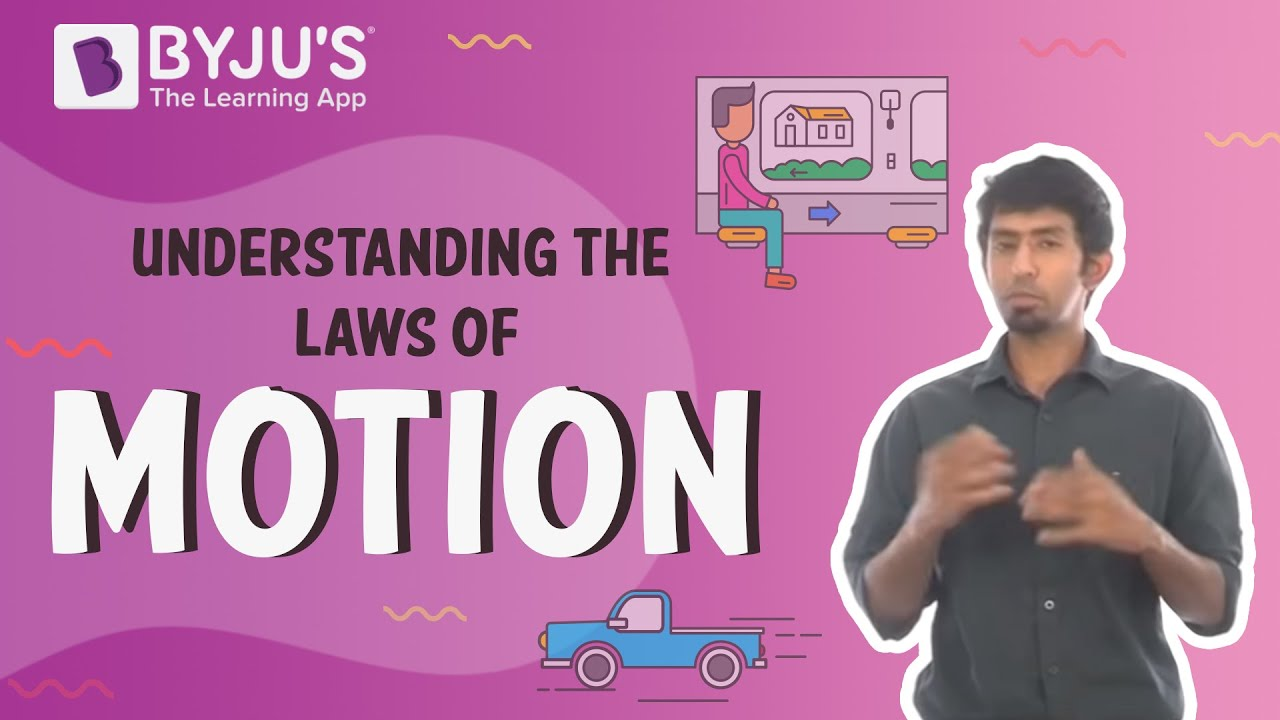# Class 11 Physics Class 11 Chapter 5 Laws of Motion MCQs

Class 11 Physics Chapter 5 Laws of Motion MCQs are provided here with answers. These questions are designed as per the latest CBSE syllabus and NCERT curriculum. Solving these chapter-wise MCQs will help students to score good marks in the final exam. Laws of Motion Class 11 Physics MCQs are prepared for a better understanding of the concept. It allows students to test their knowledge and answering skills in the given time frame.

## MCQs on Class 11 Chapter 5 Laws of Motion

Check the multiple-choice questions for the 11th Class Physics Laws of Motion chapter. Each MCQ will have four options here, out of which only one is correct. Students have to pick the correct option and check the answer provided here.

Download Chapter 5 Laws of Motion MCQs PDF by clicking on the button below.

1. What is the net force of a kite that is held stationary in the sky?

1. 1
2. Increasing
3. 0
4. Decreasing

Explanation: It is zero since the kite is at rest.

2. Which is the branch of physics that deals with the motion of a body by considering the cause?

1. Statics
2. Thermodynamics
3. Dynamics
4. Astronomy

Explanation: Dynamics deals with the motion of a body by considering the cause.

3. What is the formula to find linear velocity?

1. Linear velocity = 2(Mass + Velocity)
2. Linear velocity = Mass / Velocity
3. Linear velocity = Mass * Velocity
4. Linear velocity = Mass – Velocity

Answer: (c) Linear velocity = Mass * Velocity

Explanation: The linear momentum of a body is defined as the product of the mass of the body and its velocity.

4. Which is the type of inertia?

1. Inertia of rest
2. Inertia of motion
3. Inertia of direction
4. All the above options

Answer: (d) All the above options

Explanation: Types of inertia are: Inertia of rest, Inertia of motion, and Inertia of direction.

5. When an external force is not applied to the system, its total momentum _____________

1. Becomes zero
2. Remains constant

Explanation: We know that the total momentum of an isolated system of particles is conserved. When an external force is not applied to the system, its total momentum remains constant.

6. Apparent weight of a body = actual weight of the body, if the body _____________

1. Moves with increasing velocity
2. Remains at rest
3. Remains in a state of uniform motion
4. Option (b) and (c)

Answer: (d) Option (b) and (c)

Explanation: Apparent weight of a body is equal to its actual weight if the body is either in a state of rest or in a state of uniform motion.

7. Forces acting for a short duration are called _____________

1. Short force
2. Interval force
3. Impulsive forces
4. Interrupting force

Explanation: The force which acts on an object for a short period of time is known as Impulsive force.

8. An aircraft executes a horizontal loop at a speed of 720 km/h with its wings banked at 15°. What is the radius of the loop?

1. 15 km
2. 23.567 m
3. 12.781 m
4. 14 km

Explanation:

The angle of tilt of the aircraft is given by,

tanθ= v2/rg

Here, θ is the angle of tilt of the aircraft, r is the radius of the loop, v is the loop speed and g is the acceleration due to gravity.

Substitute the values in the above equation.

$$\begin{array}{l}r = \frac{(720 \, km/h \times \frac{1}{3600}s)^2}{(10\,m \times \frac{1}{1000}\, km) \times tan15} \approx 15\, km\end{array}$$

Thus, the radius of the loop is 15 km.

9. The inherent property, with which a body resists any change in its state of motion is known as _____________

1. Force
2. Momentum
3. Inertia
4. Acceleration

Explanation: Inertia is the inherent property, with which a body resists any change in its state of motion.

10. 1 Newton = _____________

1. 1 Newton = 1 kg x 1 m/s2
2. 1 Newton = 1 kg
3. 1 Newton = 1 kg x 1 m/s
4. 1 Newton = m/s2

Answer: (a) 1 Newton = 1 kg x 1 m/s2

## Understand the Laws of Motion and the concepts behind these theories by watching this intriguing video.